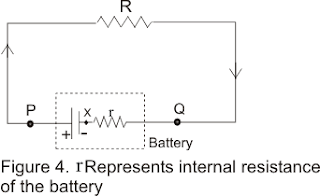# Internal Resistance of Battery (or cell)

-The resistance offered by medium in between plates of battery (electrolytes and electrodes of the cell) to the flow of current within the battery is called internal resistance of the battery.
Internal resistance of a battery usually d branch containing batteryenoted by r and in electric circuit its representation is shown below in the figure-Internal resistance of a battery depends on factors like seperation between plates, plate area, nature of material of plate etc. For an ideal cell r=0 , but real batteries or sourcesof emf always has same finite internal resistance.
-If P and Q are two terminals of the battery shown below in the figurethen potential difference between terminals P and Q is
VP=(VP-Vx) - (VQ - Vx) = E-Ir
let VP-VQ=V
V=E-IR
now for I=0 and V=EMF
and this potential difference V is called the terminal difference of the cell or battery and defined as the emf of the battery when no current drawn from it.
-For real battery equation(4) which gives V=E-Ir whereI is the current in the branch containing battery.
-From figure(4) potential difference across the external resistance R of the circuit would be equal to terminal potential difference of the cell. Thus
V=IR also V=E-Ir
or, IR=E-Ir
which gives
I=E/(R+r) =Net EMF/Net resistance
-From equation(4) we can calculate that when current is drawn from the battery terminal potential difference is less than the EMF of the battery.